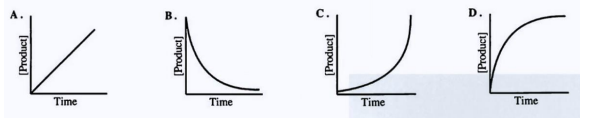# Problem: The oxidation of propane is given by the reaction below: C3H8 (g) + O2 (g) → 3 CO2 (g) + 4 H2O (l) Which graph accurately shows the concentration of CO2 as a function of the time for the reaction above?

🤓 Based on our data, we think this question is relevant for Professor Dixon's class at UCF.

###### Problem Details

The oxidation of propane is given by the reaction below:

C3H8 (g) + O2 (g) → 3 CO2 (g) + 4 H2O (l)

Which graph accurately shows the concentration of CO2 as a function of the time for the reaction above?What scientific concept do you need to know in order to solve this problem?

Our tutors have indicated that to solve this problem you will need to apply the Rate of Reaction concept. You can view video lessons to learn Rate of Reaction. Or if you need more Rate of Reaction practice, you can also practice Rate of Reaction practice problems.

What is the difficulty of this problem?

Our tutors rated the difficulty ofThe oxidation of propane is given by the reaction below: C3...as low difficulty.

How long does this problem take to solve?

Our expert Chemistry tutor, Jules took 1 minute and 57 seconds to solve this problem. You can follow their steps in the video explanation above.

What professor is this problem relevant for?

Based on our data, we think this problem is relevant for Professor Dixon's class at UCF.